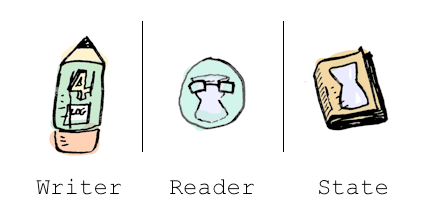Note: before reading this, you should know what a monad is. Read this post if you don't!

Here's a function half: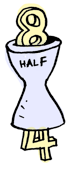And we can apply it a couple of times:

half . half $8 => 2  Everything works as expected. Now you decide that you want to log what happens in this function:half x = (x div 2, "I just halved " ++ (show x) ++ "!")  Okay, fine. Now what if you want to apply half a couple of times? half . half$ 8


Here's what we want to have happen:Spoilers: it doesn't happen automatically. You have to do it yourself:

finalValue = (val2, log1 ++ log2)
where (val1, log1) = half 8
(val2, log2) = half val1


Yuck! That's nowhere as nice as:

half . half $8  And what if you have more functions that log things? There's a pattern here: for each function that returns a log along with a value, we want to combine those logs. This is a side-effect, and monads are great at side effects! ## The Writer monadThe Writer monad is cool. "Hey dude, I'll handle the logging," says Writer. "Go back to your clean code and crank up some Zeppelin!" Every writer has a log and a return value: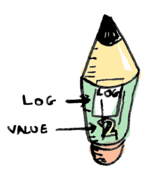data Writer w a = Writer { runWriter :: (a, w) }  Writer lets us write code like this: half 8 >>= half  Or you can use the <=< function, which does function composition with monads, to get: half <=< half$ 8


which is pretty darn close to half . half $8. Cool! You use tell to write something to the log. And return puts a value in a Writer. Here's our new half function: half :: Int -> Writer String Int half x = do tell ("I just halved " ++ (show x) ++ "!") return (x div 2)  It returns a Writer: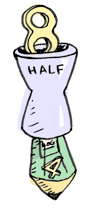And we can use runWriter to extract the values from the Writer:runWriter$ half 8
=> (4, "I just halved 8!")


But the cool part is, now we can chain calls to half with >>=:

runWriter $half 8 >>= half => (2, "I just halved 8!I just halved 4!")  Here's what's happening: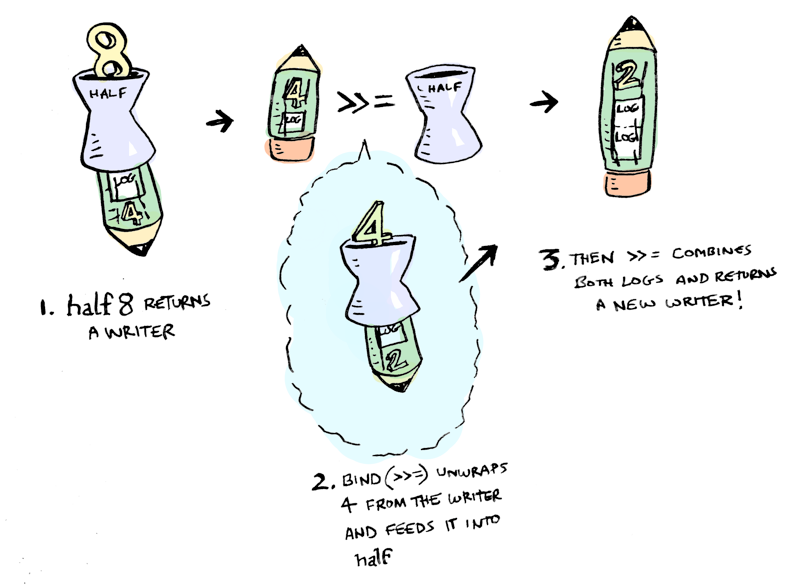>>= magically knows how to combine two writers, so we don't have to write any of that tedious code ourselves! Here's the full definition of >>=: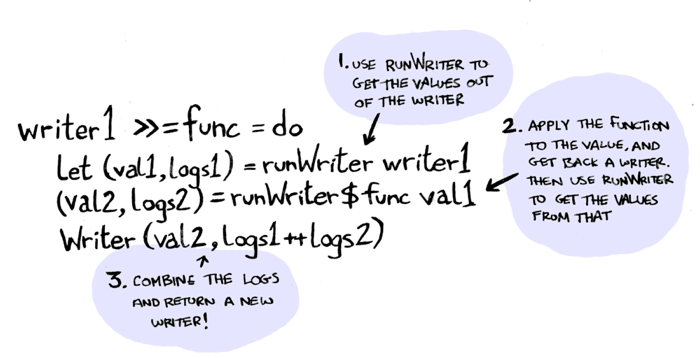Which is the same boilerplate code we had written before. Except now, >>= takes care of it for us. Cool! We also used return, which takes a value and puts it in a monad:return val = Writer (val, "")  (Note: these definitions are almost right. The real Writer monad allows us to use any Monoid as the log, not just strings. I have simplified it here a bit). Thanks, Writer monad! ## The Reader Monad Suppose you want to pass some config around to a lot of functions. Use the Reader monad:The reader monad lets you pass a value to all your functions behind the scenes. For example: greeter :: Reader String String greeter = do name <- ask return ("hello, " ++ name ++ "!")  greeter returns a Reader monad:Here's how Reader is defined: data Reader r a = Reader { runReader :: r -> a }  Reader was always the renegade. The wild card. Reader is different because it's only field is a function, and this is confusing to look at. But we both understand that you can use runReader to get that function: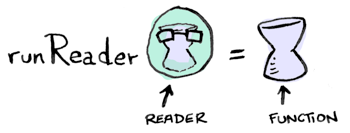And then you give this function some state, and it's used in greeter:runReader greeter$ "adit"


So when you use >>=, you should get a Reader back. When you pass in a state to that reader, it should be passed through to every function in that monad.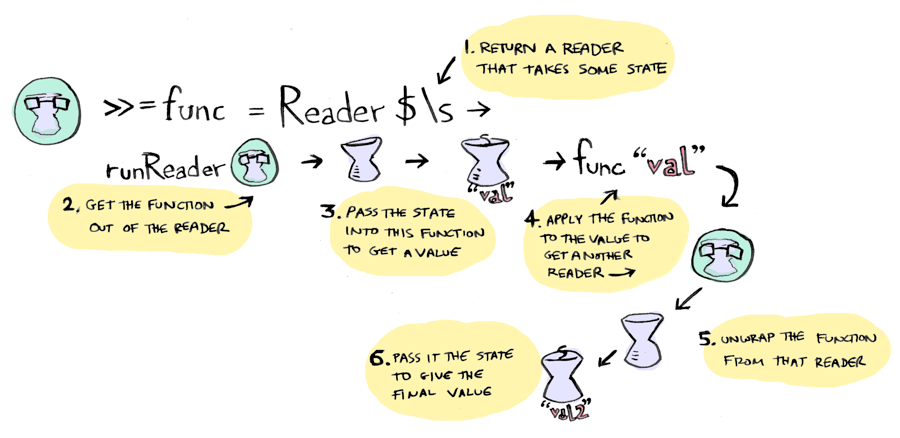m >>= k  = Reader $\r -> runReader (k (runReader m r)) r  Reader always was a little complex. The complex ones are the best. return puts a value in a Reader:return a = Reader$ \_ -> a


And finally, ask gives you back the state that was passed in:

ask = Reader $\x -> x  Want to spend some more time with Reader? Turn up the punk rock and see this longer example. ## The State Monad The State monad is the Reader monad's more impressionable best friend:She's exactly like the Reader monad, except you can write as well as read! Here's how State is defined: State s a = State { runState :: s -> (a, s) }You can get the state with get, and change it with put. Here's an example: greeter :: State String String greeter = do name <- get put "tintin" return ("hello, " ++ name ++ "!") runState greeter$ "adit"


Nice! Reader was all like "you won't change me", but State is committed to this relationship and willing to change.

The definitions for the State monad look pretty similar to the definitions for the Reader monad:

return:return a = State $\s -> (a, s)  >>=: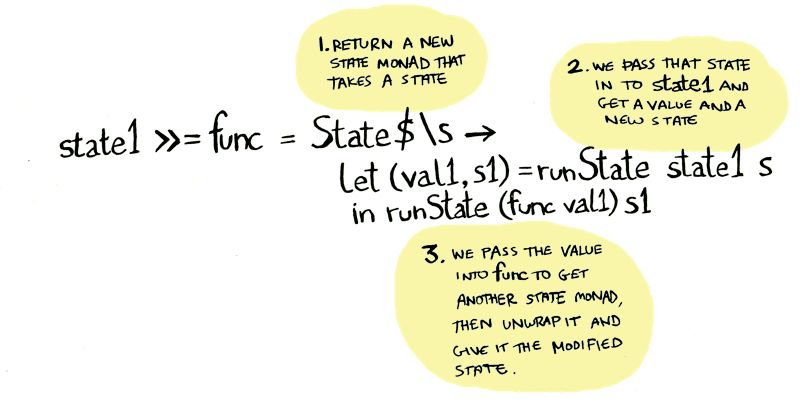m >>= k = State$ \s -> let (a, s') = runState m s
in runState (k a) s'


## Conclusion